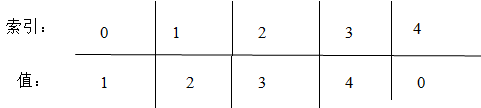## Problem

Given an array nums containing n + 1 integers where each integer is between 1 and n (inclusive), prove that at least one duplicate number must exist. Assume that there is only one duplicate number, find the duplicate one.

Note:

1. You must not modify the array (assume the array is read only).
2. You must use only constant, O(1) extra space.
3. Your runtime complexity should be less than O(n2).
4. There is only one duplicate number in the array, but it could be repeated more than once.

## Solution

### Analysis

1. 数组只读,不能修改—-不能用数组中的数字做下标,移动数组: 这样移动后nums[i] = i+1, 当nums[i] == nums[j]说明重复, 返回这个数; 同时不能使用排序
2. 常量空间—-不能使用hash表: O(n); 也不能创建新数组再排序
3. 时间复杂度小于O(N*N)—不能用循环,暴力破解
4. 只有一个数字重复,但可能重复多次(不仅一次).### Code

Solution One

Solution Two

https://www.cnblogs.com/grandyang/p/4843654.html